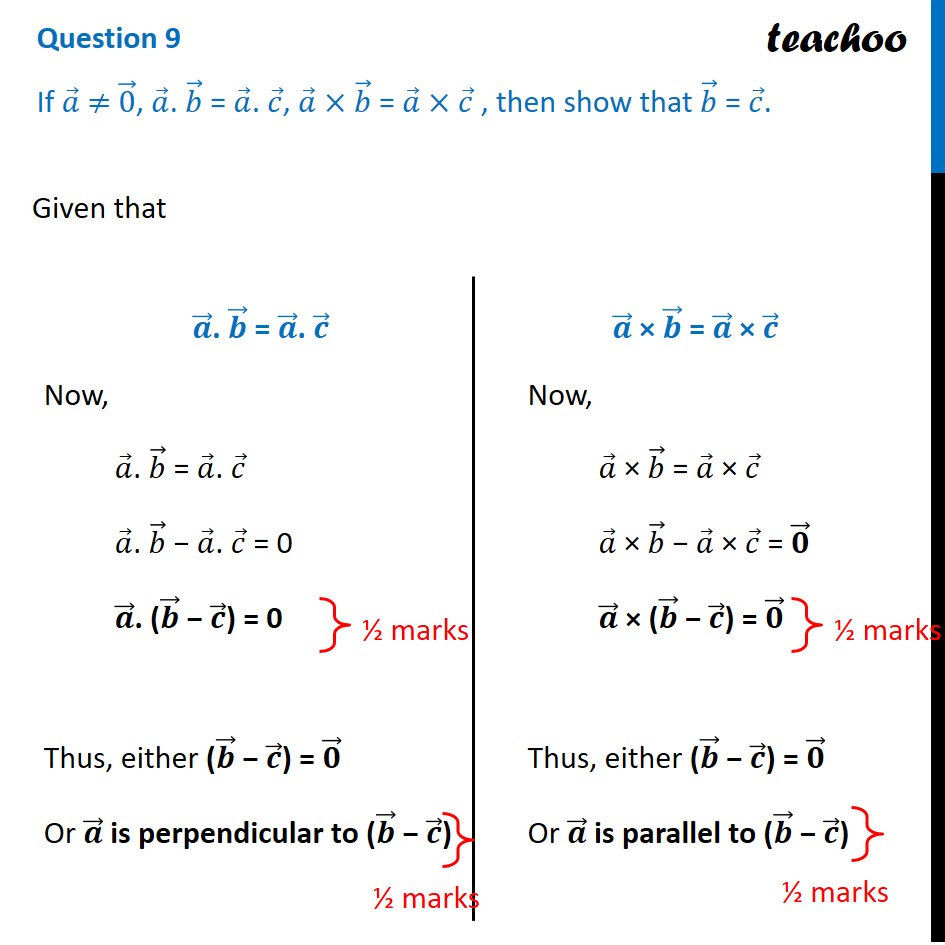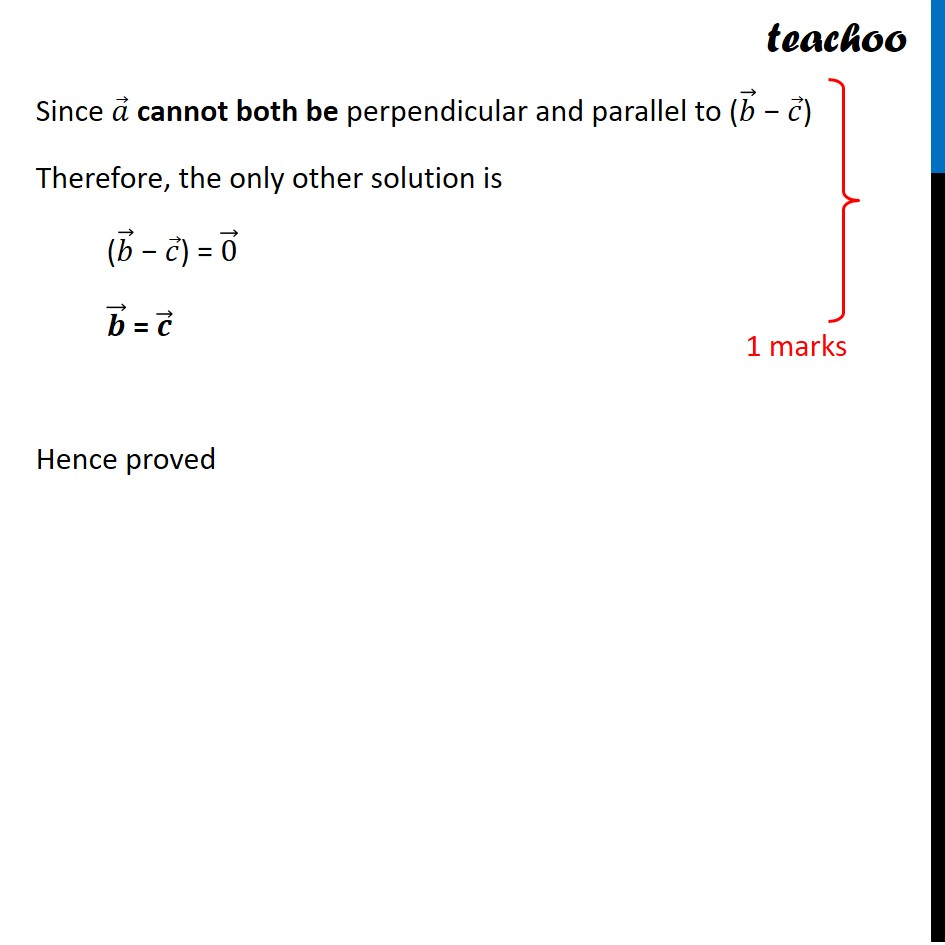CBSE Class 12 Sample Paper for 2022 Boards (For Term 2)

Class 12
Solutions of Sample Papers and Past Year Papers - for Class 12 Boards

## If 𝑎 ⃗ ≠ 0 ⃗, 𝑎 ⃗. 𝑏 ⃗ = 𝑎 ⃗. 𝑐 ⃗, 𝑎 ⃗ × 𝑏 ⃗ = 𝑎 ⃗ × 𝑐 ⃗ , then show that 𝑏 ⃗ = 𝑐 ⃗.Learn in your speed, with individual attention - Teachoo Maths 1-on-1 Class

### Transcript

Question 9 If 𝑎 ⃗ ≠ 0 ⃗, 𝑎 ⃗. 𝑏 ⃗ = 𝑎 ⃗. 𝑐 ⃗, 𝑎 ⃗ × 𝑏 ⃗ = 𝑎 ⃗ × 𝑐 ⃗ , then show that 𝑏 ⃗ = 𝑐 ⃗. Given that 𝒂 ⃗. 𝒃 ⃗ = 𝒂 ⃗. 𝒄 ⃗ Now, 𝑎 ⃗. 𝑏 ⃗ = 𝑎 ⃗. 𝑐 ⃗ 𝑎 ⃗. 𝑏 ⃗ − 𝑎 ⃗. 𝑐 ⃗ = 0 𝒂 ⃗. (𝒃 ⃗ − 𝒄 ⃗) = 0 Thus, either (𝒃 ⃗ − 𝒄 ⃗) = 𝟎 ⃗ Or 𝒂 ⃗ is perpendicular to (𝒃 ⃗ − 𝒄 ⃗) 𝒂 ⃗ × 𝒃 ⃗ = 𝒂 ⃗ × 𝒄 ⃗ Now, 𝑎 ⃗ × 𝑏 ⃗ = 𝑎 ⃗ × 𝑐 ⃗ 𝑎 ⃗ × 𝑏 ⃗ − 𝑎 ⃗ × 𝑐 ⃗ = 𝟎 ⃗ 𝒂 ⃗ × (𝒃 ⃗ − 𝒄 ⃗) = 𝟎 ⃗ Thus, either (𝒃 ⃗ − 𝒄 ⃗) = 𝟎 ⃗ Or 𝒂 ⃗ is parallel to (𝒃 ⃗ − 𝒄 ⃗) Since 𝑎 ⃗ cannot both be perpendicular and parallel to (𝑏 ⃗ − 𝑐 ⃗) Therefore, the only other solution is (𝑏 ⃗ − 𝑐 ⃗) = 0 ⃗ 𝒃 ⃗ = 𝒄 ⃗ Hence proved